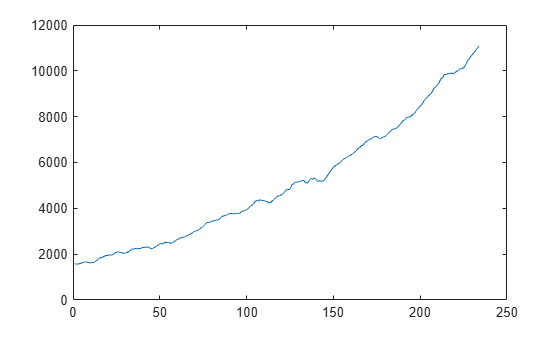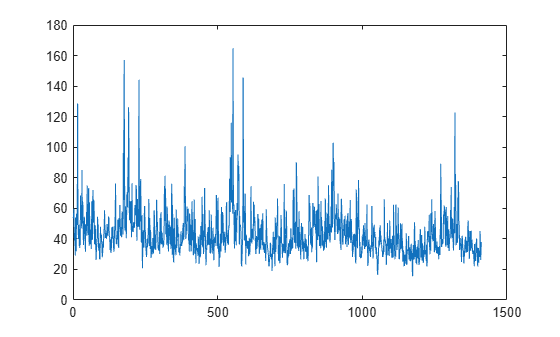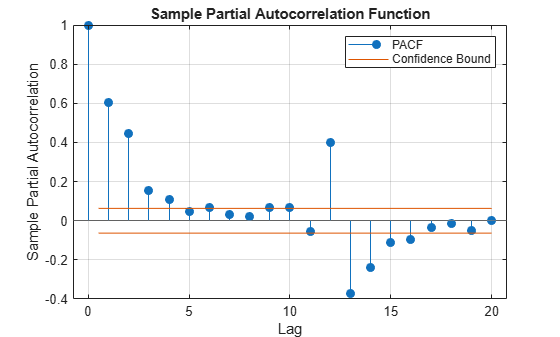# parcorr

Sample partial autocorrelation

## Syntax

``[pacf,lags] = parcorr(y)``
``PACFTbl = parcorr(Tbl)``
``````[___,bounds] = parcorr(___)``````
``[___] = parcorr(___,Name=Value)``
``parcorr(___)``
``parcorr(ax,___)``
``````[___,h] = parcorr(___)``````

## Description

example

````[pacf,lags] = parcorr(y)` returns the sample partial autocorrelation function (PACF) `pacf` and associated lags `lags` of the univariate time series `y`.```

example

````PACFTbl = parcorr(Tbl)` returns the table `PACFTbl` containing variables for the sample PACF and associated lags of the last variable in the input table or timetable `Tbl`. To select a different variable in `Tbl`, for which to compute the PACF, use the `DataVariable` name-value argument.```

example

``````[___,bounds] = parcorr(___)``` uses any input-argument combination in the previous syntaxes, and returns the output-argument combination for the corresponding input arguments and the approximate upper and lower confidence bounds `bounds` on the PACF.```

example

````[___] = parcorr(___,Name=Value)` uses additional options specified by one or more name-value arguments. For example, `parcorr(Tbl,DataVariable="RGDP",NumLags=10,NumSTD=1.96)` returns 10 lags of the sample PACF of the table variable `"RGDP"` in `Tbl` and 95% confidence bounds.```

example

````parcorr(___)` plots the sample PACF of the input series with confidence bounds.```
````parcorr(ax,___)` plots on the axes specified by `ax` instead of the current axes (`gca`). `ax` can precede any of the input argument combinations in the previous syntaxes.```
``````[___,h] = parcorr(___)``` plots the sample PACF of the input series and additionally returns handles to plotted graphics objects. Use elements of `h` to modify properties of the plot after you create it.```

## Examples

collapse all

Compute the PACF of a univariate time series. Input the time series data as a numeric vector.

Load the quarterly real GDP series in `Data_GDP.mat`. Plot the series, which is stored in the numeric vector `Data`.

```load Data_GDP plot(Data)```The series exhibits exponential growth.

Compute the returns of the series.

`ret = price2ret(Data);`

`ret` is a series of real GDP returns; it has one less observation than the real GDP series.

Compute the PACF of the real GDP returns, and return the associated lags.

```[pacf,lags] = parcorr(ret); [pacf lags]```
```ans = 21×2 1.0000 0 0.3329 1.0000 0.0828 2.0000 -0.1205 3.0000 -0.1080 4.0000 -0.0869 5.0000 0.0226 6.0000 -0.0254 7.0000 -0.0243 8.0000 0.0699 9.0000 ⋮ ```

Let ${\mathit{y}}_{\mathit{t}}$ be the real GDP return at time t. `pacf(3)` = 0.0828 means that the correlation between ${\mathit{y}}_{\mathit{t}}$ and ${\mathit{y}}_{\mathit{t}-2}$, after adjusting for the linear effects of ${\mathit{y}}_{\mathit{t}-1}$ on ${\mathit{y}}_{\mathit{t}}$, is 0.0828.

Compute the PACF of a time series, which is one variable in a table.

Load the electricity spot price data set `Data_ElectricityPrices.mat`, which contains the daily spot prices in the timetable `DataTimeTable`.

```load Data_ElectricityPrices.mat DataTimeTable.Properties.VariableNames```
```ans = 1x1 cell array {'SpotPrice'} ```

Plot the series.

`plot(DataTimeTable.SpotPrice)`The time series plot does not clearly indicate an exponential trend or unit root.

Compute the PACF of the raw spot price series.

`PACFTbl = parcorr(DataTimeTable)`
```PACFTbl=21×2 table Lags PACF ____ ________ 0 1 1 0.5541 2 0.10938 3 0.099833 4 0.029511 5 0.038836 6 0.065892 7 0.029965 8 0.034951 9 0.050091 10 0.051031 11 0.033994 12 0.051877 13 0.028973 14 0.047456 15 0.11895 ⋮ ```

`parcorr` returns the results in the table `PACFTbl`, where variables correspond to the PACF (`PACF`) and associated lags `(Lags)`.

By default, `parcorr` computes the PACF of the last variable in the table. To select a variable from an input table, set the `DataVariable` option.

Consider the electricity spot prices in Compute PACF of Table Variable.

Load the electricity spot price data set `Data_ElectricityPrices.mat`. Compute the PACF and return the PACF confidence bounds.

```load Data_ElectricityPrices [PACFTbl,bounds] = parcorr(DataTimeTable)```
```PACFTbl=21×2 table Lags PACF ____ ________ 0 1 1 0.5541 2 0.10938 3 0.099833 4 0.029511 5 0.038836 6 0.065892 7 0.029965 8 0.034951 9 0.050091 10 0.051031 11 0.033994 12 0.051877 13 0.028973 14 0.047456 15 0.11895 ⋮ ```
```bounds = 2×1 0.0532 -0.0532 ```

Assuming the spot prices follow a Gaussian white noise series, an approximate 95.4% confidence interval on the PACF is (-0.0532, 0.0532).

Load the US quarterly macroeconomic series data `Data_USEconModel.mat`. Remove all missing values from the timetable of data `DataTimeTable` by using listwise deletion.

```load Data_USEconModel DataTimeTable = rmmissing(DataTimeTable);```

Compute the PACF of the raw effective federal funds rate `FEDFUNDS` by using the OLS method (the default method when the data does not contain any missing values). Change the name of the `PACF` variable of the output table to `ols`.

```PACFTbl = parcorr(DataTimeTable,DataVariable="FEDFUNDS",Method="ols"); PACFTbl = renamevars(PACFTbl,"PACF","ols");```

Compute the PACF of the raw effective federal funds rate `FEDFUNDS` by solving the Yule-Walker equations. Store the result as the variable `yw` in `PACFTbl`.

`PACFTbl.yw = parcorr(DataTimeTable.FEDFUNDS,Method="yule-walker");`

Compare the PACFs between the methods.

`PACFTbl`
```PACFTbl=21×3 table Lags ols yw ____ _________ _________ 0 1 1 1 0.92881 0.91502 2 0.074551 0.061337 3 0.14949 0.15031 4 -0.23745 -0.20808 5 0.073346 0.073911 6 -0.25854 -0.21128 7 0.021783 0.018625 8 0.10817 0.092159 9 0.16147 0.10987 10 -0.081344 -0.077452 11 0.050378 0.034769 12 0.075574 0.070003 13 0.026074 0.02403 14 -0.036989 -0.025924 15 0.074656 0.048657 ⋮ ```

Plot the PACFs in the same stem plot.

```stem(repmat(PACFTbl.Lags,1,2),PACFTbl{:,["ols" "yw"]},"filled") title("PACF of Effective Federal Funds Rate") legend(["OLS" "Yule-Walker"])```Specify the AR(2) model:

`${y}_{t}=0.6{y}_{t-1}-0.5{y}_{t-2}+{\epsilon }_{t},$`

where ${\epsilon }_{t}$ is Gaussian with mean 0 and variance 1.

```rng(1); % For reproducibility Mdl = arima(AR={0.6 -0.5},Constant=0,Variance=1)```
```Mdl = arima with properties: Description: "ARIMA(2,0,0) Model (Gaussian Distribution)" SeriesName: "Y" Distribution: Name = "Gaussian" P: 2 D: 0 Q: 0 Constant: 0 AR: {0.6 -0.5} at lags [1 2] SAR: {} MA: {} SMA: {} Seasonality: 0 Beta: [1×0] Variance: 1 ```

Simulate 1000 observations from `Mdl`.

`y = simulate(Mdl,1000);`

Plot the PACF. Specify that the series is an AR(2) process.

`parcorr(y,NumAR=2)`The PACF cuts off after the second lag. This behavior indicates an AR(2) process.

Specify the multiplicative seasonal ARMA $\left(2,0,1\right)×\left(3,0,0{\right)}_{12}$ model:

`$\left(1-0.75L-0.15{L}^{2}\right)\left(1-0.9{L}^{12}+0.75{L}^{24}-0.5{L}^{36}\right){y}_{t}=2+{\epsilon }_{t}-0.5{\epsilon }_{t-1},$`

where ${\epsilon }_{t}$ is Gaussian with mean 0 and variance 1.

```Mdl = arima(AR={0.75 0.15},SAR={0.9 -0.75 0.5}, ... SARLags=[12 24 36],MA=-0.5,Constant=2, ... Variance=1);```

Simulate data from `Mdl`.

```rng(1); y = simulate(Mdl,1000); ```

Plot the default partial autocorrelation function (PACF).

```figure parcorr(y)```The default correlogram does not display the dependence structure for higher lags.

Plot the PACF for 40 lags.

```figure parcorr(y,NumLags=40)```The correlogram shows the larger correlations at lags 12, 24, and 36.

## Input Arguments

collapse all

Observed univariate time series for which `parcorr` computes or plots the PACF, specified as a numeric vector.

Data Types: `double`

Time series data, specified as a table or timetable. Each row of `Tbl` contains contemporaneous observations of all variables.

Specify a single series (variable) by using the `DataVariable` argument. The selected variable must be numeric.

Axes on which to plot, specified as an `Axes` object.

By default, `parcorr` plots to the current axes (`gca`).

Note

Specify missing observations using `NaN`. The `parcorr` function treats missing values as missing completely at random.

### Name-Value Arguments

Specify optional pairs of arguments as `Name1=Value1,...,NameN=ValueN`, where `Name` is the argument name and `Value` is the corresponding value. Name-value arguments must appear after other arguments, but the order of the pairs does not matter.

Before R2021a, use commas to separate each name and value, and enclose `Name` in quotes.

Example: `parcorr(Tbl,DataVariable="RGDP",NumLags=10,NumSTD=3)` plots `10` lags of the sample PACF of the variable `"RGDP"` in `Tbl`, and displays confidence bounds consisting of `3` standard errors away from 0.

Number of lags in the sample PACF, specified as a positive integer. `parcorr` uses lags `0:NumLags` to estimate the PACF.

The default is `min([20,T – 1])`, where `T` is the effective sample size of the input time series.

Example: `parcorr(y,Numlags=10)` plots the sample PACF of `y` for lags `0` through `10`.

Data Types: `double`

Number of lags in a theoretical AR model of the input time series, specified as a nonnegative integer less than `NumLags`.

`parcorr` uses `NumAR` to estimate confidence bounds. For lags > `NumAR`, `parcorr` assumes that the input times series is a Gaussian white noise process. Consequently, the standard error is approximately $1/\sqrt{T},$ where T is the effective sample size of the input time series.

Example: `parcorr(y,NumAR=10)` specifies that `y` is an AR(`10`) process and plots confidence bounds for all lags greater than `10`.

Data Types: `double`

Number of standard errors in the confidence bounds, specified as a nonnegative scalar. For all lags greater than `NumAR`, the confidence bounds are 0 ± `NumSTD*`$\stackrel{^}{\sigma }$, where $\stackrel{^}{\sigma }$ is the estimated standard error of the sample partial autocorrelation.

The default yields approximate 95% confidence bounds.

Example: `parcorr(y,NumSTD=1.5)` plots the PACF of `y` with confidence bounds `1.5` standard errors away from 0.

Data Types: `double`

PACF estimation method, specified as a value in this table.

ValueDescriptionRestrictions
`"ols"`Ordinary least squares (OLS)The input times series must be fully observed (it cannot contain any `NaN` values).
`"yule-walker"`Yule-Walker equationsNone.

If the input time series is fully observed, the default is `"ols"`. Otherwise, the default is `"yule-walker"`.

Example: `parcorr(y,Method="yule-walker")` computes the PACF of `y` using the Yule-Walker equations.

Data Types: `char` | `string`

Variable in `Tbl` for which `parcorr` computes the PACF, specified as a string scalar or character vector containing a variable name in `Tbl.Properties.VariableNames`, or an integer or logical vector representing the index of a name. The selected variable must be numeric.

Example: `DataVariable="GDP"`

Example: `DataVariable=[false true false false]` or `DataVariable=2` selects the second table variable.

Data Types: `double` | `logical` | `char` | `string`

## Output Arguments

collapse all

Sample PACF, returned as a numeric vector of length `NumLags` + `1`. `parcorr` returns `pacf` only when you supply the input `y`.

The elements of `pacf` correspond to lags 0, 1, 2, ..., `NumLags` (that is, elements of `lags`). For all time series, the lag 0 partial autocorrelation `pacf(1)` = `1`.

PACF lags, returned as a numeric vector with elements `0:NumLags`. `parcorr` returns `lags` only when you supply the input `y`.

Sample PACF, returned as a table with variables for the outputs `pacf` and `lags`. `parcorr` returns `PACFTbl` only when you supply the input `Tbl`.

Approximate upper and lower confidence bounds assuming the input series is an AR(`NumAR`) process, returned as a two-element numeric vector. The `NumSTD` option specifies the number of standard errors from 0 in the confidence bounds.

Handles to plotted graphics objects, returned as a graphics array. `h` contains unique plot identifiers, which you can use to query or modify properties of the plot.

collapse all

### Partial Autocorrelation Function

The partial autocorrelation function measures the correlation between yt and yt + k after adjusting for the linear effects of yt + 1,...,yt + k – 1.

The estimation of the PACF involves solving the Yule-Walker equations with respect to the autocorrelations. However, if the time series is fully observed, then the PACF can be estimated by fitting successive autoregressive models of orders 1, 2, ... using ordinary least squares. For details, see , Chapter 3.

### Missing Completely at Random

Observations of a random variable are missing completely at random if the tendency of an observation to be missing is independent of both the random variable and the tendency of all other observations to be missing.

## Tips

• To plot the PACF without confidence bounds, set `NumSTD=0`.

## Algorithms

`parcorr` plots the PACF when you do not request any output or when you request the fourth output `h`.

 Box, George E. P., Gwilym M. Jenkins, and Gregory C. Reinsel. Time Series Analysis: Forecasting and Control. 3rd ed. Englewood Cliffs, NJ: Prentice Hall, 1994.

 Hamilton, James D. Time Series Analysis. Princeton, NJ: Princeton University Press, 1994.